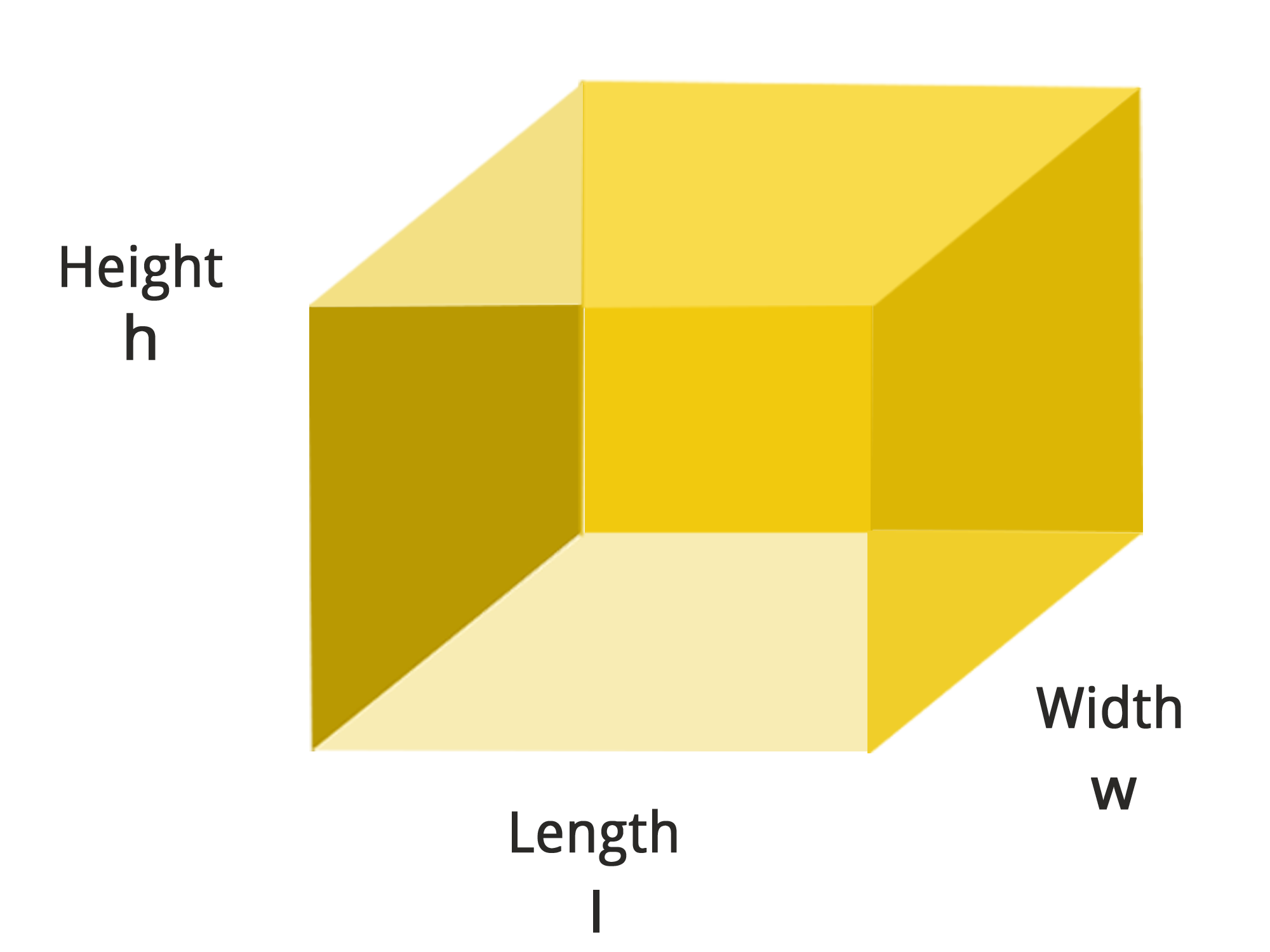• Call Now

1800-102-2727•

# Perfect Cube

The perfect cube is the result of multiplying the same integer three times. Multiplying the number 4 three times, for example, yields 64. As a result, the number 64 is a perfect cube. Perfect cube = number x number x number. If a number can be broken down into a product of the same three numbers, it is said to be a perfect cube.## Definition of a Perfect Cube

A perfect cube is the product of three identical numbers. To see if a number 'N' is a perfect cube, multiply it by itself three times and see if the result is the number 'N'. If you answered yes, you have a perfect cube. A perfect cube has the numbers 1, 8, 27, and 64 as examples. A perfect square can be made by multiplying two numbers together. It's not the same as a flawless cube. Both positive and negative integers can be used to make perfect cubes. For example, -64 is a perfect cube since it is the result of multiplying -4 three times.

## Root Cube

When we say a number has been cubed, we indicate that it has been multiplied three times. The process of cubing a number is reversed with cube root. When the number 5 is cubed, for example, we obtain 5 x 5 x 5, which equals 125. The number 125 has a cube root of 5. This is because multiplying the number 5 three times yields 125. Cube root is represented by the symbol: It's the same as the square root sign, except with the addition of a '3' to indicate that it's a cube root. The cube root of an integer can be represented in exponent form as (number)1/3.

Number Multiplication Fact Perfect Cube
1 1 × 1 × 1 1
2 2 × 2 × 2 8
3 3 × 3 × 3 27
4 4 × 4 × 4 64
5 5 × 5 × 5 125
6 6 × 6 × 6 216
7 7 × 7 × 7 343
8 8 × 8 × 8 512
9 9 × 9 × 9 729
10 10 × 10 × 10 1000
11 11 × 11 × 11 1331
12 12 × 12 × 12 1728
13 13 × 13 × 13 2197
14 14 × 14 × 14 2744
15 15 × 15 × 15 3375
16 16 × 16 × 16 4096
17 17 × 17 × 17 4913
18 18 × 18 × 18 5832
19 19 × 19 × 19 6859
20 20 × 20 × 20 8000
21 21 × 21 × 21 9261
22 22 × 22 × 22 10648
23 23 × 23 × 23 12167
24 24 × 24 × 24 13824
25 25 × 25 × 25 15625
26 26 × 26 × 26 17576
27 27 × 27 × 27 19683
28 28 × 28 × 28 21952
29 29 × 29 × 29 24389
30 30 × 30 × 30 27000
31 31 × 31 × 31 29791
32 32 × 32 × 32 32768
33 33 × 33 × 33 35937
34 34 × 34 × 34 39304
35 35 × 35 × 35 42875
36 36 × 36 × 36 46656
37 37 × 37 × 37 50653
28 38 × 38 × 38 54872
39 39 × 39 × 39 59319
40 40 × 40 × 40 64000
41 41 × 41 × 41 68921
42 42 × 42 × 42 74088
43 43 × 43 × 43 79507
44 44 × 44 × 44 85184
45 45 × 45 × 45 91125
46 46 × 46 × 46 97336
47 47 × 47 × 47 103823
48 48 × 48 × 48 110592
49 49 × 49 × 49 117649
50 50 × 50 × 50 125000

There is a method for determining whether or not larger numbers are perfect cubes. To check, add up all of the digits in the number and determine if the result is 0, 1, 8, or 9. If it's any of these, it may be a perfect cube, although that isn't always the case. To determine if it is a perfect cube, we must repeat the prime factorization procedure described before. This is a method for determining if a number is a perfect cube or not, however it is not always guaranteed.

This technique is utilized because let's assume we're given a number, like 8768, and we're not sure if it's a perfect cube or not. So, we calculate the sum of this number's digits and see if it's 0, 1, 8, or 9. If the number isn't one of these four, we don't need to factorize it to see if it's a perfect cube. In other words, if the sum is not equal to 0, 1, 8, or 9, this technique just bypasses the prime factorization procedure. In this example, the total of the digits of the integers 8768 equals 29, the sum of 2 and 9 is 11, and 1 + 1 = 2. As a result, the answer isn't 0, 1, 8, or 9. As a result, it's easy to deduce that 8768 isn't a perfect cube.Talk to our expert
Resend OTP Timer =
By submitting up, I agree to receive all the Whatsapp communication on my registered number and Aakash terms and conditions and privacy policy